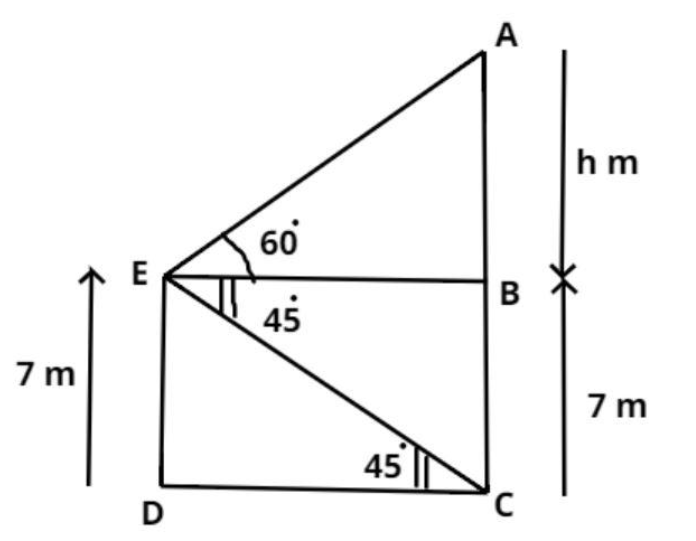Courses
Courses for Kids
Free study material
Free LIVE classes
MoreLIVE
Join Vedantu’s FREE Mastercalss

# From the top of a 7 m high building, the angle of elevation of the top of a cable tower is 60 degree and the angle of depression of its foot is 45 degree. The height of the tower in meter is $A.{\text{ }}7\left( {\sqrt 3 - 1} \right) \\ B.{\text{ }}7\sqrt 3 \\ C.{\text{ }}7 + \sqrt 3 \\ D.{\text{ }}7\left( {\sqrt 3 + 1} \right) \\$Verified
269.7k+ views
Hint: In this question first draw the pictorial representation of the problem it will give us a clear picture of what we have to calculate later on apply the property of tan which is the ratio of perpendicular to base so use these concepts to reach the solution of the question.Let us consider ED to be a building.
Let us consider ED to be a building.
$\Rightarrow E D=7$ meter (given)
Let us consider AC to be the cable tower.
And it is given that the angle of elevation of the top of a cable tower from the top of a $7 \mathrm{~m}$ high building is 60
degree and the angle of depression of its foot is 45 degree.
$\Rightarrow \angle B E A=60^{0}, \angle B E C=45^{0}$ (see figure)
Now by symmetry $E D=B C=7$ meter and $E B=D C$ (see figure)
So the height $(\mathrm{H})$ of the cable tower is $(\mathrm{AB}+\mathrm{BC})$.
Let $A B$ be $h$ meter.
$\Rightarrow H=h+7$ meter.................. (1)
Now first calculate EB.
So, in triangle EBC
$\Rightarrow \tan 45^{0}=\dfrac{\text { Perpendicular }}{\text { Base }}=\dfrac{B C}{E B}=\dfrac{7}{E B}$
Now as we know that the value of tan 45 degree is 1
$\Rightarrow E B=7$ meter.
Now in triangle ABE
$\Rightarrow \tan 60^{\circ}=\dfrac{\text { Perpendicular }}{\text { Base }}=\dfrac{A B}{E B}=\dfrac{h}{7}$
And as we know that the value of $\tan 60^{0}=\sqrt{3}$ so, substitute this value in above equation we have,
$\Rightarrow \sqrt{3}=\dfrac{h}{7}$
$\Rightarrow h=7 \sqrt{3}$ meter.
Now from equation (1) we have,
$\Rightarrow H=h+7=7 \sqrt{3}+7=7(\sqrt{3}+1)$ meter.
So, the height of the cable tower is $7(\sqrt{3}+1)$ meter,
Hence option (d) is correct.

Note: In such types of questions pictorial representation will give us the idea of what we have to calculate then remember the property of tan which is stated above and calculate the length of EB and AB respectively after calculating these values the height of the cable tower is the sum of length AB and BC so add these and simplify, we will get the required length of the cable tower which is the required answer.
Last updated date: 21st Sep 2023
Total views: 269.7k
Views today: 7.69k